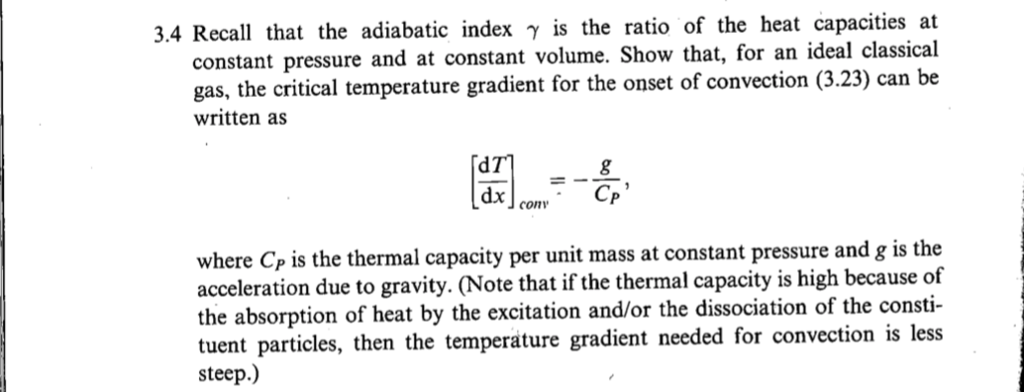# .4 Recall that the adiabatic index is the ratio of the heat capacities at constant pressure...

###### Question:.4 Recall that the adiabatic index is the ratio of the heat capacities at constant pressure and at constant volume. Show that, for an ideal classical gas, the critical temperature gradient for the onset of convection (3.23) can be written as dx con where Cp is the thermal capacity per unit mass at constant pressure and g is the acceleration due to gravity. (Note that if the thermal capacity is high because of the absorption of heat by the excitation and/or the dissociation of the consti- tuent particles, then the temperature gradient needed for convection is less steep.)

#### Similar Solved Questions

##### Only C. note the check figure for the entry says 6,000 cannot get that amount. CHAPTER...
only C. note the check figure for the entry says 6,000 cannot get that amount. CHAPTER 11 Partnerships ALTERNATE PROBLEMS Problem 11-1B Methods of allocating partnership profit LO3 Xcel CHECK FIGURE: C. Dr. Lister, Capital: $6,000 Phung, Moier, and Lister invested$130,000, $150,000, and$120,000...
##### Let X, Y be independent random variables with E[X] = E[Y] = 0 and ox =...
Let X, Y be independent random variables with E[X] = E[Y] = 0 and ox = oy = 5. Then Var(2x +3Y) = 1. True False...
##### When we do scientific study we A. Read scientific articles B. Find out explanations with absolute...
When we do scientific study we A. Read scientific articles B. Find out explanations with absolute certainty C. Collect Data D. Understand natural process with the possibility of change E. Conduct surveys...